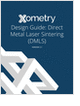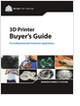Formula HomeMechanics of MaterialsStressStrainHooke's LawApplicationsPressure VesselsRosette Strain GagesFailure CriteriaCalculatorsStress TransformStrain TransformPrincipal StressPrincipal StrainElastic ConstantsResourcesBibliography

Learn the best principles to negotiate the salary you deserve!

Metal 3D Printing Design Guide

Direct Metal Laser Sintering (DMLS) 3D printing for parts with reduced cost and little waste.

STEM Career Outlook

Wages, employment opportunities, and growth projections for STEM jobs.

Selecting the Right 3D Printer

Discover how to choose the right 3D printer for your needs and the key performance attributes to consider.

more free publicationsCalculator IntroductionFor isotropic materials, only two independent elastic constants are needed for describing the stress-strain relationship, i.e., Hooke's Law. This calculator computes the inter-relations among the 5 commonly used elastic constants:
 Symbol Name Other Names E Youngs modulus Elastic modulus, tension modulus n Poisson ratio G or m Shear modulus Rigidity modulus, 2nd Lamé constant K Bulk modulus Compression modulus l 1st Lamé constant
Select 2 constants for input, and calculate the rest.

 E: Young's Modulus nu: Poisson's Ratio G: Shear Modulus K: Bulk Modulus lambda: Lamé's constant E: Young's Modulus nu: Poisson's Ratio G: Shear Modulus K: Bulk Modulus lambda: Lamé's constant Units for modulii (E, G, K, l) Pa kPa MPa GPa psi ksi

 Young's Modulus E: 70.00 GPa Poisson's Ratio n: 0.30 Shear Modulus G: 26.92 GPa Bulk Modulus K: 58.33 GPa Lamé's Constant l: 40.38 GPa

Table of Relations among Elastic ConstantsThe relationships amongst the 5 elastic constants are shown in the table below.

 InputConstants Output Relations E = n = G = K = l = E, n - -E, G --E, K --E, l -- n, G- -n, K--n, l-- G, K- -G, l-- K, l- -

For the case of {E, l} input, the factor R is defined as,The 2nd Law of Thermodynamics requires that the following limits hold on the elastic constants,

E, G, and K > 0GlossaryLearn the best principles to negotiate the salary you deserve!Metal 3D Printing Design Guide

Direct Metal Laser Sintering (DMLS) 3D printing for parts with reduced cost and little waste.STEM Career Outlook

Wages, employment opportunities, and growth projections for STEM jobs.Selecting the Right 3D Printer

Discover how to choose the right 3D printer for your needs and the key performance attributes to consider.